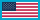Change Location# Learning and Experiencing Cryptography with CrypTool and SageMath

By (author):
Pages: 420
ISBN: 9781685690175
Coming Soon: Available 12/31/2023

Hardback \$169.00 Qty:

This book provides a broad overview of cryptography and enables cryptography for trying out. It emphasizes the connections between theory and practice, focuses on RSA for introducing number theory and PKI, and links the theory to the most current recommendations from NIST and BSI. The book also enables readers to directly try out the results with existing tools available as open source. It is different from all existing books because it shows very concretely how to execute many procedures with different tools. The target group could be self-learners, pupils and students, but also developers and users in companies. All code written with these open-source tools is available. The appendix describes in detail how to use these tools. The main chapters are independent from one another. At the end of most chapters, you will find references and web links. The sections have been enriched with many footnotes. Within the footnotes you can see where the described functions can be called and tried within the different CrypTool versions, within SageMath or within OpenSSL.

Introduction

1 Ciphers and Attacks against Them
1.1 Importance of cryptology
1.2 Symmetric encryption
1.3 Asymmetric encryption
1.4 Hybrid procedures
1.5 Kerckhoffs’ principle
1.6 Key spaces – theoretical and practical view
1.7 Attack types and security definitions
1.8 Best known attacks on given ciphers
1.9 Algorithm types and self-made ciphers
1.10 Further references / Recommended books
1.11 AES visualizations/implementations
1.12 Educational examples for symmetric ciphers using SageMath

2 P&P and Pre-Computer Ciphers
2.1 Transposition ciphers 2.2 Substitution ciphers
2.3 Combining substitution and transposition
2.4 Further P&P methods (including new ones)
2.5 Hagelin machines as sample for pre-computer ciphers
2.6 Ciphers defined by ACA
2.7 Samples of open-access publications on cracking classical ciphers
2.8 Examples using SageMath

3 Historical Cryptology
3.1 Introduction
3.2 Analyzing historical ciphers – from collection to interpretation
3.3 Collection of manuscripts and creation of metadata
3.4 Transcription
3.5 Cryptanalysis
3.6 Contextualization and interpretation: Historical and philological analysis
3.7 Conclusion

4 Prime Numbers
4.1 What are prime numbers?
4.2 Prime numbers in mathematics
4.3 How many prime numbers are there?
4.4 The search for extremely large primes
4.5 Prime number tests
4.6 Special types of numbers and the search for a formula for primes
4.7 Density and distribution of the primes
4.8 Outlook
4.10 Number of prime numbers in various intervals
4.11 Indexing prime numbers (n-th prime number)
4.12 Orders of magnitude / dimensions in reality
4.13 Special values of the binary and decimal system
4.14 Visualization of the quantity of primes in higher ranges
4.15 Examples using SageMath

5 Introduction to Elementary Number Theory with Examples
5.1 Mathematics and cryptography
5.2 Introduction to number theory
5.3 Prime numbers and the first fundamental theorem of elementary number theory
5.4 Divisibility, modulus and remainder classes
5.5 Calculations with finite sets
5.6 Examples of modular calculations
5.7 Groups and modular arithmetic in Zn and Z
5.8 Euler function, Fermat’s little theorem and Euler-Fermat
5.9 Multiplicative order and primitive roots
5.10 Proof of the RSA procedure with Euler-Fermat
5.11 Security aspects regarding the security of practical RSA implementations
5.12 Considerations regarding the security of the RSA algorithm
5.13 Applications of asymmetric cryptography using numerical examples
5.14 The RSA procedure with actual numbers
5.15 Didactic comments on modulo subtraction
5.16 Base representation of numbers, estimation of length of digits
5.17 Examples using SageMath

6 The Mathematical Ideas behind Modern (Asymmetric) Cryptography
6.1 One way functions with trapdoor and complexity classes
6.2 Knapsack problem as a basis for public-key procedures
6.3 Decomposition into prime factors as a basis for public-key procedures
6.4 The discrete logarithm as basis for public-key procedures
6.5 The RSA plane
6.6 Outlook

7 Hash Functions, Digital Signatures, and PKIs
7.1 Hash functions
7.2 Digital signatures
7.3 RSA signatures
7.4 DSA signatures
7.5 Public-key certification

8 Elliptic-Curve Cryptography (ECC)
8.1 Elliptic-curve cryptography – a high-performance substitute for RSA?
8.2 Elliptic curves – history
8.3 Elliptic curves – mathematical basics
8.4 Elliptic curves in cryptography
8.5 Operating on the elliptic curve
8.6 Security of elliptic-curve cryptography: the ECDLP
8.7 Encryption and signing with elliptic curves
8.8 Factorization using elliptic curves
8.9 Implementing elliptic curves for educational purposes
8.10 Patent aspects
8.11 Elliptic curves in use

9 Foundations of Modern Symmetric Encryption
9.1 Boolean functions
9.2 Block ciphers
9.3 Stream ciphers
9.4 Table of SageMath examples in this chapter

10 Homomorphic Ciphers
10.1 Origin of the term “homomorphic”
10.2 Decryption function is a homomorphism
10.3 Classification of homomorphic methods
10.4 Examples of homomorphic pre-FHE ciphers
10.5 Applications
10.6 Homomorphic methods in CrypTool

11 Lightweight Introduction to Lattices
11.1 Preliminaries
11.2 Equations
11.3 Systems of linear equations
11.4 Matrices
11.5 Vectors
11.6 Equations – revisited
11.7 Vector spaces
11.8 Lattices
11.9 Lattices and RSA
11.10 Lattice basis reduction
11.11 PQC standardization

12 Solving Discrete Logarithms and Factoring
12.1 Generic algorithms for the discrete logarithm problem in any group
12.2 Best algorithms for prime fields Fp
12.3 Best known algorithms for extension fields Fpn and recent advances
12.4 Best known algorithms for factoring integers
12.5 Best known algorithms for elliptic curves
12.6 Possibility of embedded backdoors in cryptographic keys
12.7 Conclusion: Advice for cryptographic infrastructure

13 Future Use of Cryptography
13.1 Widely used schemes
13.2 Preparing for tomorrow
13.3 New mathematical problems
13.4 New signatures
13.5 Quantum cryptography – a way out of the dead end?
13.6 Post-Quantum Cryptography (PQC)

13.7 Conclusion

• Bernhard Esslinger